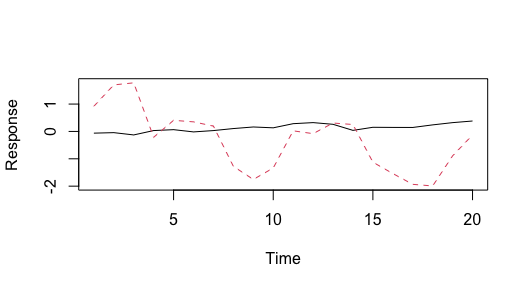# Estimating process trend variability with bayesdfa

#### 2021-09-28

A constraint of most DFA models is that the latent trends are modeled as random walks with fixed standard deviations (= 1). We can evaluate the ability to estimate these parameters in a Bayesian context below.

library(bayesdfa)
library(ggplot2)
library(dplyr)
library(rstan)
chains = 1
iter = 10

## Case 1: unequal trend variability

First, let’s simulate some data. We will use the built-in function sim_dfa(), but normally you would start with your own data. We will simulate 20 data points from 4 time series, generated from 2 latent processes. For this first dataset, the data won’t include extremes, and loadings will be randomly assigned (default).

set.seed(1)
sim_dat <- sim_dfa(
num_trends = 2,
num_years = 20,
num_ts = 4
)

We’ll tweak the default code though to model slightly different random walks, with different standard deviations for each trend. We’ll assume these standard deviations are 0.3 and 0.5, respectively.

set.seed(1)
sim_dat$x[1,] = cumsum(rnorm(n=ncol(sim_dat$x), 0, 0.1))
sim_dat$x[2,] = cumsum(rnorm(n=ncol(sim_dat$x), 0, 1))

Looking at the random walks, we can see the 2nd trend (red dashed line) is more variable than the black line.

matplot(t(sim_dat$x), type = "l", ylab = "Response", xlab = "Time" )Simulated data, from a model with 2 latent trends and no extremes. Next, we’ll calculate the predicted values of each time series and add observation error sim_dat$pred = sim_dat$Z %*% sim_dat$x
for(i in 1:nrow(sim_dat$pred)) { for(j in 1:ncol(sim_dat$pred)) {
sim_dat$y_sim[i,j] = sim_dat$pred[i,j] + rnorm(1,0,0.1)
}
}

### Candidate models

Next, we’ll fit a 2-trend DFA model to the simulated time series using the fit_dfa() function. This default model fixed the variances of the trends at 1 – and implicitly assumes that they have equal variance.

f1 <- fit_dfa(
y = sim_dat$y_sim, num_trends = 2, scale="zscore", iter = iter, chains = chains, thin = 1 ) r1 <- rotate_trends(f1) Next, we’ll fit the model with process errors being estimated – and assume unequal variances by trend. f2 <- fit_dfa( y = sim_dat$y_sim, num_trends = 2, scale="zscore", estimate_process_sigma = TRUE,
equal_process_sigma = FALSE,
iter = iter, chains = chains, thin = 1
)
r2 <- rotate_trends(f2)

Third, we’ll fit the model with process errors being estimated, unequal variances by trend, and not z-score the data (this is just a test of the scaling).

f3 <- fit_dfa(
y = sim_dat$y_sim, num_trends = 2, scale="center", estimate_process_sigma = TRUE, equal_process_sigma = FALSE, iter = iter, chains = chains, thin = 1 ) r3 <- rotate_trends(f3) Our fourth and fifth models will be the same as # 2 and # 3, but will estimate a single variance for both trends. f4 <- fit_dfa( y = sim_dat$y_sim, num_trends = 2, scale="zscore", estimate_process_sigma = TRUE,
equal_process_sigma = TRUE,
iter = iter, chains = chains, thin = 1
)
r4 <- rotate_trends(f4)

f5 <- fit_dfa(
y = sim_dat$y_sim, num_trends = 2, scale="center", estimate_process_sigma = TRUE, equal_process_sigma = TRUE, iter = iter, chains = chains, thin = 1 ) r5 <- rotate_trends(f5) ### Recovering loadings As a reminder, let’s look at the loadings from the original simulation model print(round(sim_dat$Z,2))
##       [,1]  [,2]
## [1,]  0.57  0.00
## [2,] -0.14  0.33
## [3,]  1.18  1.06
## [4,] -1.52 -0.30

The estimated loadings from the DFA where the trends are forced to have the same fixed variance are good

print(round(r1$Z_rot_mean,2)) ## [,1] [,2] ## [1,] -91.70 -32.20 ## [2,] -10.27 -109.07 ## [3,] 42.34 -12.66 ## [4,] -28.00 -7.90 but some of the loadings are far off. These loadings are also not well estimated for either of the models that estimate the process variances, print(round(r2$Z_rot_mean,2))
##        [,1]    [,2]
## [1,] -70.20   68.36
## [2,] -14.61 -118.64
## [3,] -66.64    9.32
## [4,] -47.34  -10.62

or

print(round(r3$Z_rot_mean,2)) ## [,1] [,2] ## [1,] -92.62 37.23 ## [2,] -100.07 -64.45 ## [3,] -3.13 -44.88 ## [4,] -83.12 -29.19 The loadings for Model 4 are given by print(round(r4$Z_rot_mean,2))
##        [,1]   [,2]
## [1,] -99.51   5.65
## [2,]  -7.46 -98.49
## [3,]   8.32   2.71
## [4,]   1.16   0.06

and Model 5 by

print(round(r5\$Z_rot_mean,2))
##        [,1]    [,2]
## [1,] -89.54  -26.98
## [2,] -19.58 -107.16
## [3,]  44.17   66.35
## [4,] -47.99  -90.43

If we calculate the RMSE of the different models, model # 3 (estimated process trends, raw data not standardized) performs the best